Question

Q.16 A ball of mass 1200 gm is thrown up vertically with an initial velocity of...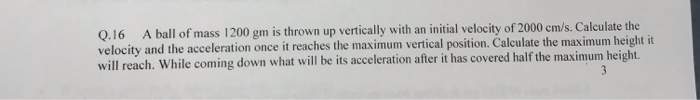Q.16 A ball of mass 1200 gm is thrown up vertically with an initial velocity of 2000 cm/s. Calculate the velocity and the acceleration once it reaches the maximum vertical position. Calculate the maximum height it will reach. While coming down what will be its acceleration after it has covered half the maximum height.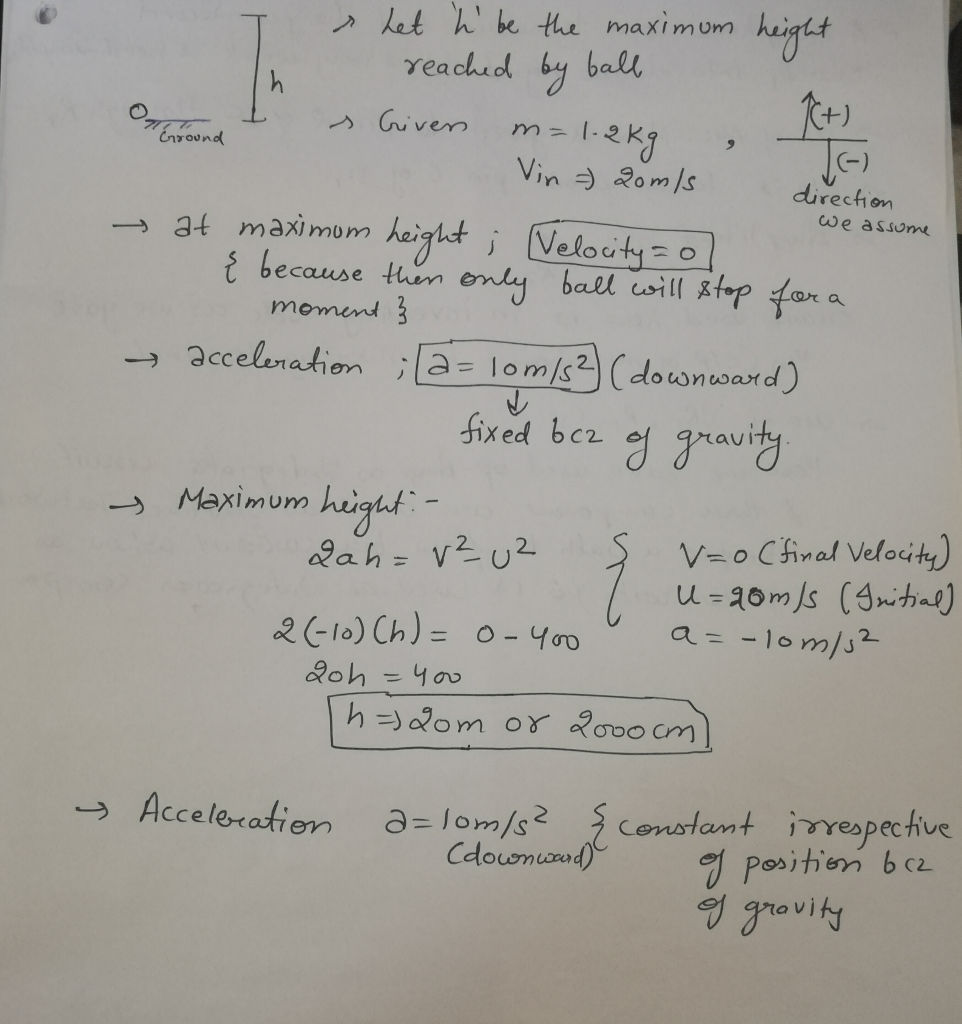Earn Coins

Coins can be redeemed for fabulous gifts.

Similar Homework Help Questions
• 3. A ball is thrown straight up, reaches a maximum height, then falls to its initial...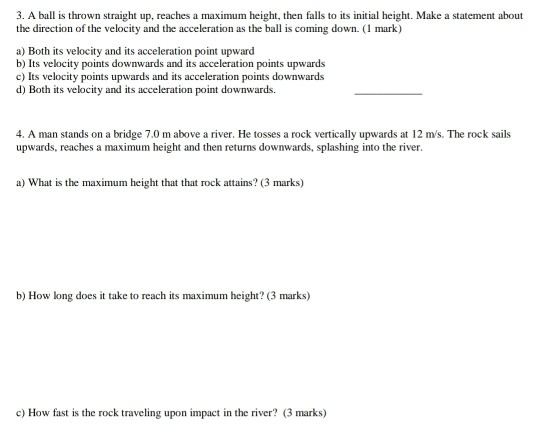3. A ball is thrown straight up, reaches a maximum height, then falls to its initial height. Make a statement about the direction of the velocity and the acceleration as the ball is coming down. (1 mark) a) Both its velocity and its acceleration point upward b) Its velocity points downwards and its acceleration points upwards c) Its velocity points upwards and its acceleration points downwards d) Both its velocity and its acceleration point downwards. 4. A man stands on...

• 3. A body of mass m is thrown vertically up with an initial velocity vo. The...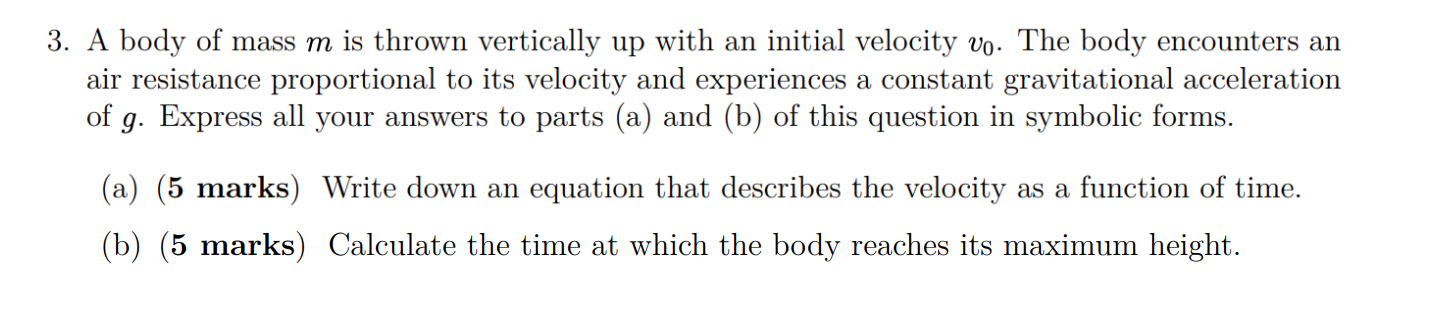3. A body of mass m is thrown vertically up with an initial velocity vo. The body encounters an air resistance proportional to its velocity and experiences a constant gravitational acceleration of g. Express all your answers to parts (a) and (b) of this question in symbolic forms. (a) (5 marks) Write down an equation that describes the velocity as a function of time. (b) (5 marks) Calculate the time at which the body reaches its maximum height.

• A ball is thrown vertically upwards with an initial velocity of 18.80 m/s, from the ground....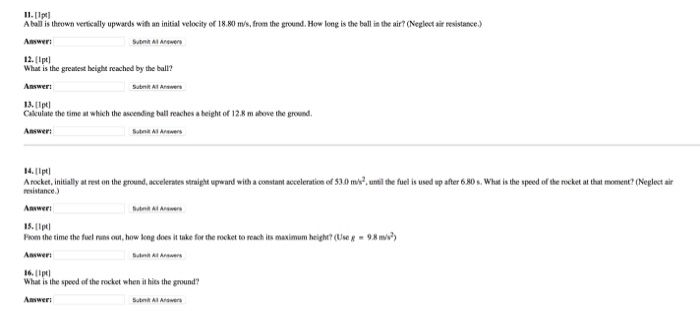A ball is thrown vertically upwards with an initial velocity of 18.80 m/s, from the ground. How long is the ball in the air? (Neglect air resistance.) What is the greatest height reached by the ball? Calculate the time at which the ascending ball reaches a height of 12.8 m above the ground. A rocket, initially at rest on the ground, accelerates straight upward with a constant acceleration of 53.0 m/s^2, until the fuel is used up after 6.80s. What...

• A ball is thrown from the top of a building with an initial velocity of 23.7...

A ball is thrown from the top of a building with an initial velocity of 23.7 m/s straight upward, at an initial height of 52.0 m above the ground. The ball just misses the edge of the roof on its way down, as shown in the figure. (a) Determine the time needed for the ball to reach its maximum height. s (b) Determine the maximum height. m (c) Determine the time needed for the ball to return to the height...

• Two Thrown Balls 1 2 3 45678 A blue ball is thrown upward with an initial...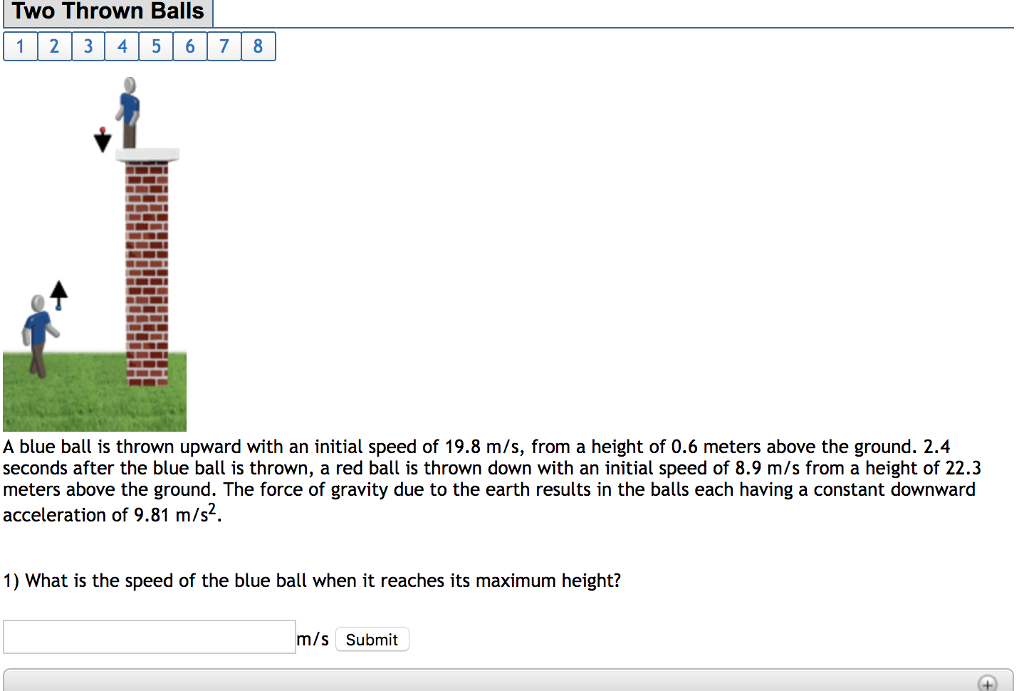Two Thrown Balls 1 2 3 45678 A blue ball is thrown upward with an initial speed of 19.8 m/s, from a height of 0.6 meters above the ground. 2.4 seconds after the blue ball is thrown, a red ball is thrown down with an initial speed of 8.9 m/s from a height of 22.3 meters above the ground. The force of gravity due to the earth results in the bals each having a constant downward acceleration of 9.81 m/s2....

• A 3.00 kg ball is thrown vertically into the air with an initial velocity of 15.0 m/s

A 3.00 kg ball is thrown vertically into the air with an initial velocity of 15.0 m/s. The maximum height of the ball is

• A ball is thrown vertically upwards with an initial velocity of 27.0 m/s. Neglecting air resistance,...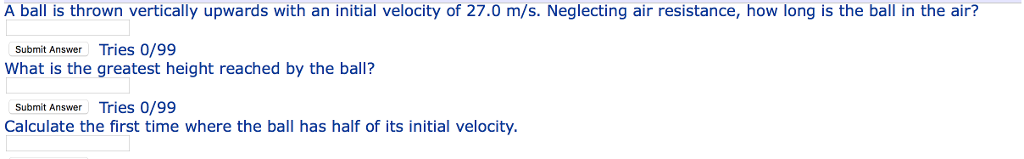A ball is thrown vertically upwards with an initial velocity of 27.0 m/s. Neglecting air resistance, how long is the ball in the air? Submit Answer Tries 0/99 What is the greatest height reached by the ball? Submit Answer Tries 0/99 Calculate the first time where the ball has half of its initial velocity

• A ball thrown vertically upward is caught by the thrower after 3.00 s

A ball thrown vertically upward is caught by the thrower after 3.00 s. Find the following:The initial velocity of the ball?The maximum height the ball reaches?

• Two throw A blue ball is thrown upward with an initial speed of 22.2 m/s, from...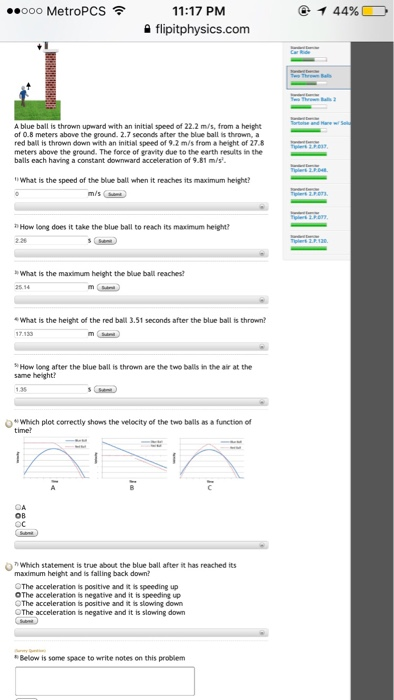Two throw A blue ball is thrown upward with an initial speed of 22.2 m/s, from a height of 0.8 meters above the ground. 2.7 seconds after the blue ball is thrown, a red ball is thrown down with an initial speed of 9.2 m/s from a height of 27.8 meters above the ground. The force of grandly due to the earth results in the balls each having a constant downward acceleration of 9.81 m/s^2. What is the speed of...

• A basketball is thrown vertically upward with an initial velocity of 22.8 m/s. 'a) How long...

A basketball is thrown vertically upward with an initial velocity of 22.8 m/s. 'a) How long (s) does the ball take to reach its maximum height? 'b) How high (km) does the basketball go?